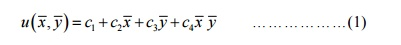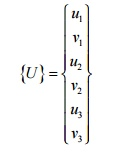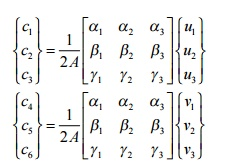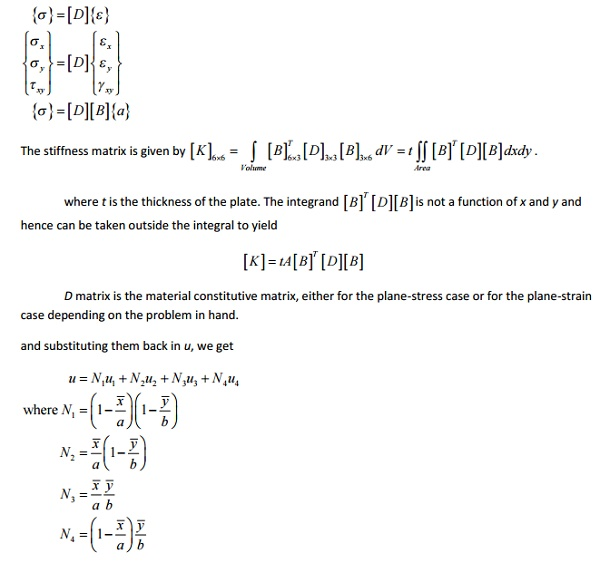Home | | Finite Element Analysis | 2,3 Noded Linear Triangular Element

# 2,3 Noded Linear Triangular Element

Three Noded Linear Triangular Element, Four Noded Linear Rectangular Element, Two-Variable 3-Noded Linear Triangular Element.

THREE NODED LINEAR TRIANGULAR ELEMENT

The physical domain considered is geometrically a 2-Dimensional domain, i.e., an area with uniform thickness and the single variable can be one of pressure, temperature, etc. (a scalar quantity, not a vector quantity). An example is the temperature distribution in a plate. At each point there can be only one temperature. We consider such an area meshed with triangular elements. Each triangular element has three nodes, (i.e., one node at each corner). Let us consider one such element with coordinates one such element with coordinates     (x1,y1), (x2,y2), (x3,y3. The single variable (for example, temperature) at these nodes 1, 2 and 3 are u1 , u 2 and u3 , respectively. If so, then the unknown single variable u (temperature) at any non-nodal point x, y in the 2-D domain can be expressed in terms of the known nodal variables (temperatures) u1 , u 2  and u3.

Let us assume that the single variable can be expressed as

U= c1+c2x+c3y

In order to find the three unknowns c1 , c2  and c3 , we apply the boundary conditionsFOUR NODED LINEAR RECTANGULAR ELEMENTLet us assume that the single variable can be expressed asThis polynomial contains four linearly independent terms and is linear in x and y, with a bilinear term in x and y. The polynomial requires an element with four nodes. There are two possible geometric shapes: a triangle with the fourth node at the centroid of the triangle or a rectangle with nodes at the vertices.

A triangle with a fourth node at the center does not provide a single-valued variation of u at inter-element boundaries, resulting in incompatible variation of u at inter-element boundaries and is therefore not admissible.

The linear rectangular element is a compatible element because on any side, the single variable u varies only linearly and there are two nodes to uniquely define it.

Here we consider an approximation of the form given in eqauation (1) and use a rectangular element with sides a and b. For the sake of convenience we choose a local coordinate system

(x, y  to derive the interpolation functions.In order to find the three unknowns c1 , c2  and c3 , we apply the boundary conditionsTWO-VARIABLE 3-NODED LINEAR TRIANGULAR ELEMENTFigure shows a 2-D two-variable linear triangular element with three nodes and the two dof at each node. The nodes are placed at the corners of the triangle. The two variables (dof) are displacement in x-direction (u) and displacement in y-direction (v). Since each node has two dof, a single element has 6 dof. The nodal displacement vector is given byWe select a linear displacement function for each dof asThe above two algebraic equations can also be written asUsing steps we had developed for the 2-D single-variable linear triangular element, we can writeand using the interpolation functions we had developed for the 2-D single-variable linear triangular element, we can write{}=[]{a}

strains associated with the two-dimensional element are given byStudy Material, Lecturing Notes, Assignment, Reference, Wiki description explanation, brief detail
Mechanical : Finite Element Analysis : Two Dimensional Finite Element Analysis : 2,3 Noded Linear Triangular Element |

Related Topics